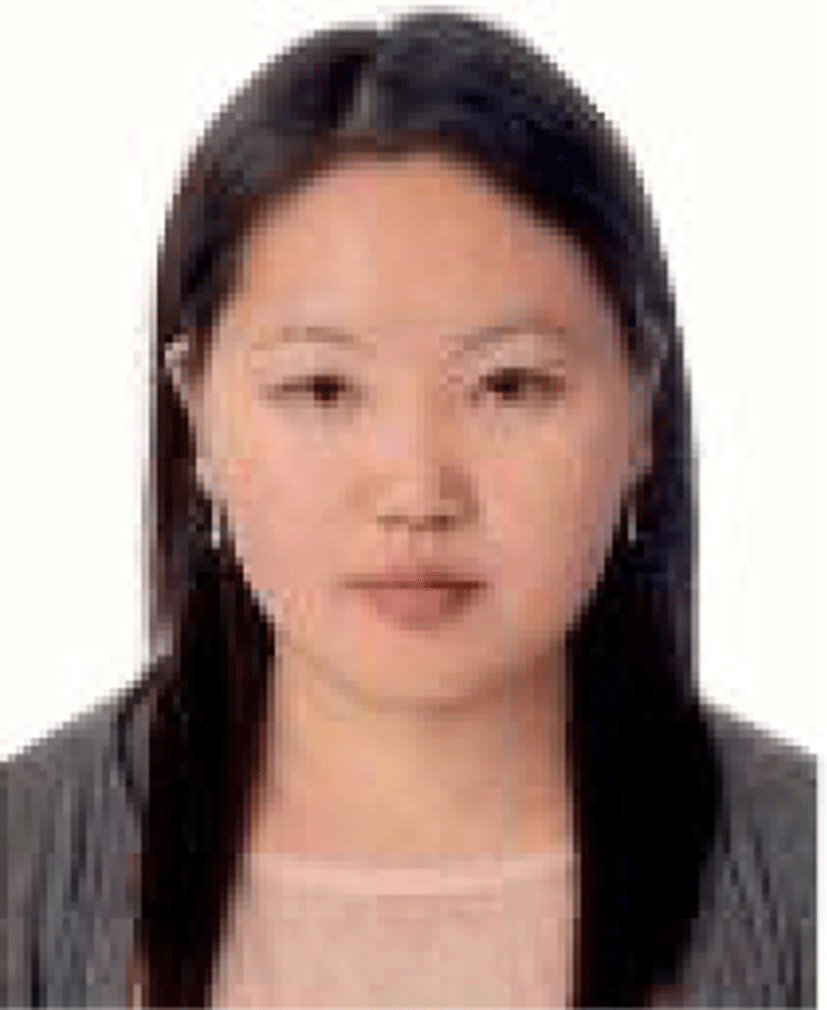Section A

# An Experimental Study of Image Thresholding Based on Refined Histogram using Distinction Neighborhood Metrics

Nyamlkhagva Sengee1, Dalaijargal Purevsuren1,*, Tserennadmid tumurbaatar1
1Department of Information and Computer Sciences, School of Engineering and Applied Sciences, National University of Mongolia, Ulaanbaatar, Mongolia, nyamlkhagva@num.edu.mn, dalaijargal@seas.num.edu.mn, tserennadmid@seas.num.edu.mn
*Corresponding Author: Dalaijargal Purevsuren, +976-88005204, dalaijargal@seas.num.edu.mn

© Copyright 2022 Korea Multimedia Society. This is an Open-Access article distributed under the terms of the Creative Commons Attribution Non-Commercial License (http://creativecommons.org/licenses/by-nc/4.0/) which permits unrestricted non-commercial use, distribution, and reproduction in any medium, provided the original work is properly cited.

Received: May 18, 2022; Revised: May 31, 2022; Accepted: Jun 01, 2022

Published Online: Jun 30, 2022

## Abstract

In this study, we aimed to illustrate that the thresholding method gives different results when tested on the original and the refined histograms. We use the global thresholding method, the well-known image segmentation method for separating objects and background from the image, and the refined histogram is created by the neighborhood distinction metric. If the original histogram of an image has some large bins which occupy the most density of whole intensity distribution, it is a problem for global methods such as segmentation and contrast enhancement. We refined the histogram to overcome the big bin problem in which sub-bins are created from big bins based on distinction metric. We suggest the refined histogram for preprocessing of thresholding in order to reduce the big bin problem. In the test, we use Otsu and median-based thresholding techniques and experimental results prove that their results on the refined histograms are more effective compared with the original ones.

Keywords: Refined Histogram; Histogram Thresholding; Neighborhood Metric

## I. INTRODUCTION

Within image segmentation techniques, thresholding methods are a simple and effective technique. Thresholding is commonly used to separate the object and background of an image and to distinguish important information in the image. Thresholding techniques do that the images are cut in two based on the selected threshold value in which greater intensities are classified as one and lower of that are into other. Although many segmentation methods are developed to segment the object of the image, thresholding techniques are very low computational cost compared with other methods. As mentioned, the thresholding idea is obvious, but the problems are the selection of threshold values. The threshold selection methods are mainly based on a histogram of images, and the techniques are suggested in many research works [1-3]. There are many thresholding techniques such as histogram shape-based, cluster-based, entropy-based, spatial-based, network-based, etc [4-5]. In this work, we suggest the refined histogram extension for popular cluster-based methods to order to reduce big bin problems.

Generally, the thresholding techniques are divided into two main groups: global and local thresholding. Global thresholding uses the histogram of the whole image to select the threshold value. In local thresholding, images are separated by different regions and they are independently selected by the threshold values in those regions .

If the histogram has some large bins which occupy the most density of whole intensity distribution, it is a problem for global thresholding methods. We refined the histogram to overcome the big bin problem in which sub-bins are created from big-bins based on distinction metric . Median-based and Otsu thresholding methods are used in our work, and those thresholding methods [8-9] are common techniques. In order to define the threshold value in Otsu thresholding, intra-class variances are calculated for every possible deal, and then the threshold value is determined at minimum intra-class variance. If the histogram of an image has two peaks relating to objects and background, the Otsu thresholding result is much better than others. However, if two peaks of the histogram have some big bins, there is still a problem in selecting the optimal threshold value. Our experimental results show that they work more effectively on the refined histograms.

Our work is organized in the following sections, and thresholding basics are discussed in section II. The refined histogram is described in section III, and Section IV shows some results implemented on original and modified histograms. Our conclusions are in Section V.

## II. THRESHOLDING TECHNIQUES

Assume that an image f(x, y) has M×N pixels and an intensity range is 0 < f(x, y) ≤ 255, x ∈ [0, M-1], and y ∈ [0, N-1]. A threshold t is used for images into two classes:

(1)

The histogram intensity levels of the image f (x,y) are (i note 0 < iL-1), and the histogram denotes h(i).

2.1. Otsu Thresholding

The idea of Otsu thresholding is to find an optimal threshold value based on minimizing intra-class variance which is calculated for every possible threshold value.

Variances of two classes are noted by σ2bg and σ2fg, and defined by:

(2)

where, Nbg and Nfg are numbers of the pixels, and ubg and ufg are means of two classes, respectively. Means are calculated by:

(3)

Intra-class variance is computed by

${\sigma }_{w}^{2}={W}_{bg}{\sigma }_{bg}^{2}+{W}_{fg}{\sigma }_{fg}^{2},$
(4)

where wbg and wfg are the weight of two classes and computed by:

(5)

To find the optimal threshold value, the Otsu algorithm is expressed by that we do the following steps for each threshold value, iteratively (step 1 to step 4):

1. Divide into two classes based on the threshold value t.

2. Calculate the weights of each class (equation 5).

3. Estimate the means of each class (equation 3).

4. Compute the intra-class variance of two classes (equation 4).

5. Select the optimal threshold based on minimizing intra-class variances.

## III. REFINED HISTOGRAM

3.1. Distinction Neighborhood Metric

In order to provide neighborhoods of all pixels of f(x, y), let g(x, y) be the extended image that is surrounded m times by zero intensities:

${g}_{m}\left(x,y\right)=\left\{\begin{array}{l}f\left(x,y\right),\left(x,y\right)\in \left[0,M-1\right]×\left[0,N-1\right]\hfill \\ 0,\text{\hspace{0.17em}}\text{\hspace{0.17em}}\text{otherwise}\hfill \end{array}.$
(6)

We proposed the distinction metric in our previous work  and the distinction metric is computed by the cumulative difference between current pixel intensity and its neighboring pixel’s intensities. It is expressed by the following formula:

$D={\sum }_{\left(x\text{'},y\text{'}\right)\in {R}_{m}\left(x,y\right)}\left(f\left(x,y\right)-R\left(x\text{'},y\text{'}\right)\right),$
(7)

where D is the distinction metric computed by equation (7) and Rm(x, y) is a local square in the m by m square neighborhood centered on f(x, y).

Based on the distinction metric, we can divide one big bin into many sub-bins. For instance, in Fig. 1, we can see that the intensity (i=30) of the image occurs in high density in its histogram. However, they may have different neighbors. Fig. 1 shows the demonstration of the big bin dividing into the sub-bins process.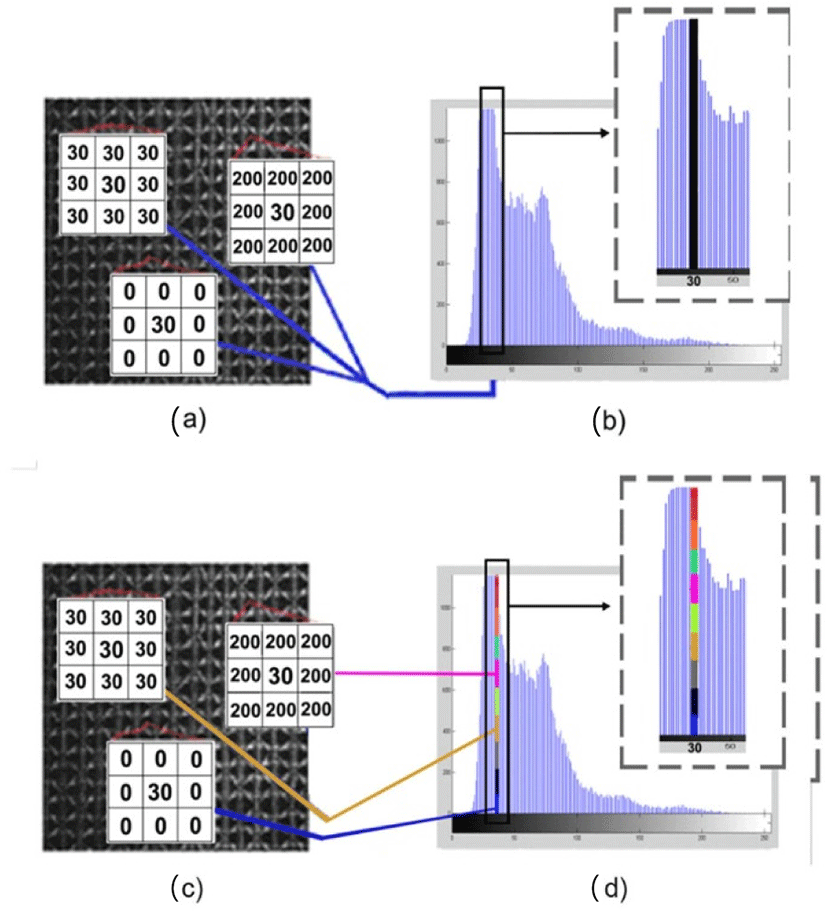Fig. 1. Demonstration of the idea of dividing one big bin into many sub-bins. (a) and (b) show the original image and its histogram; (c) and (d) show the original image and one big bin (i=30) divided into sub-bins.

## IV. EXPERIMENTAL RESULTS

This work aimed to show that one thresholding method gives different results when tested on the original and the refined histograms. In the experimental, we used six images: two images are chosen from the Brodatz texture dataset , both of them are selected from DIBCO (2019) datasets , and others are used . All selected image histograms have some big bins in which the densities of the histogram are high. Otsu and median threshold selections are used for segmenting foreground from background in those images for comparison.

In order to evaluate effectiveness, we use intersection over union (IoU) criteria, and it is expressed by the following:

$IoU=\frac{A\cap B}{A\cup B},$
(8)

where, A is the resulting image and B is the correctly binarized image (ground truth).

The experimental results show that the segmentation result used in the refined histogram is much better than that of the original. In Table 1, we can see more detailed pieces of information on the comparison.

Table 1. Original and refined histograms bin sizes and selected threshold values of them by thresholding methods, and IoU criteria values are shown.
Methods Median Otsu
Test images\Types of histogram Original Refined Original Refined
Image 1
DIBCO
2019 track A
Bin number 256 24,330 256 24,330
Threshold T= 112 12,165 188 14,966
IoU 0.94 0.92 0.91 0.88
Image 2
DIBCO
2019 track A
Bin number 256 26,658 256 26,658
Threshold T= 123 13,329 113 15,393
IoU 0.23 0.96 0.97 0.94
Image 3
Brodatz
(#D34)
Bin number 256 5,792 256 5,792
Threshold T= 122 2,896 104 1,972
IoU 0.23 0.68 0.29 0.87
Image 4
Brodatz
(#D42)
Bin number 256 15,192 256 15,192
Threshold T= 118 7,596 131 6,449
IoU 0.49 0.58 0.49 0.66
Image 5 Bin number 256 6,583 256 6,583
Threshold T= 111 3,291 68 2,276
IoU 0.26 0.51 0.64 0.75
Image 6 Bin number 256 12,193 256 12,193
Threshold T= 128 6,096 97 4,958
IoU 0.67 0.83 0.93 0.94

In Table. 1, we can see histogram bin sizes and temporary bin sizes of refined histograms in the Bin number rows. Threshold (T=) row shows selected thresholding values of the original and refined histograms for each method, respectively. IoU row is their values of evaluation metric.

Fig. 2 shows the results of median thresholding on two test images in track A of the DIBCO-2019 dataset, and their ground truth mask images are shown in Fig. 2(c) and (d). In Fig. 2(e) and (f) result images are tested on the original histograms, and (h) and (i) result images are tested on the refined histograms. In Fig. 2(e), the texts are not clearly shown while the texts are clearly shown in (h). By contrast, background texts are more segmented with proposed texts on the original histogram in Fig. 2(f), and it was reduced on the refined histogram in Fig. 2(i).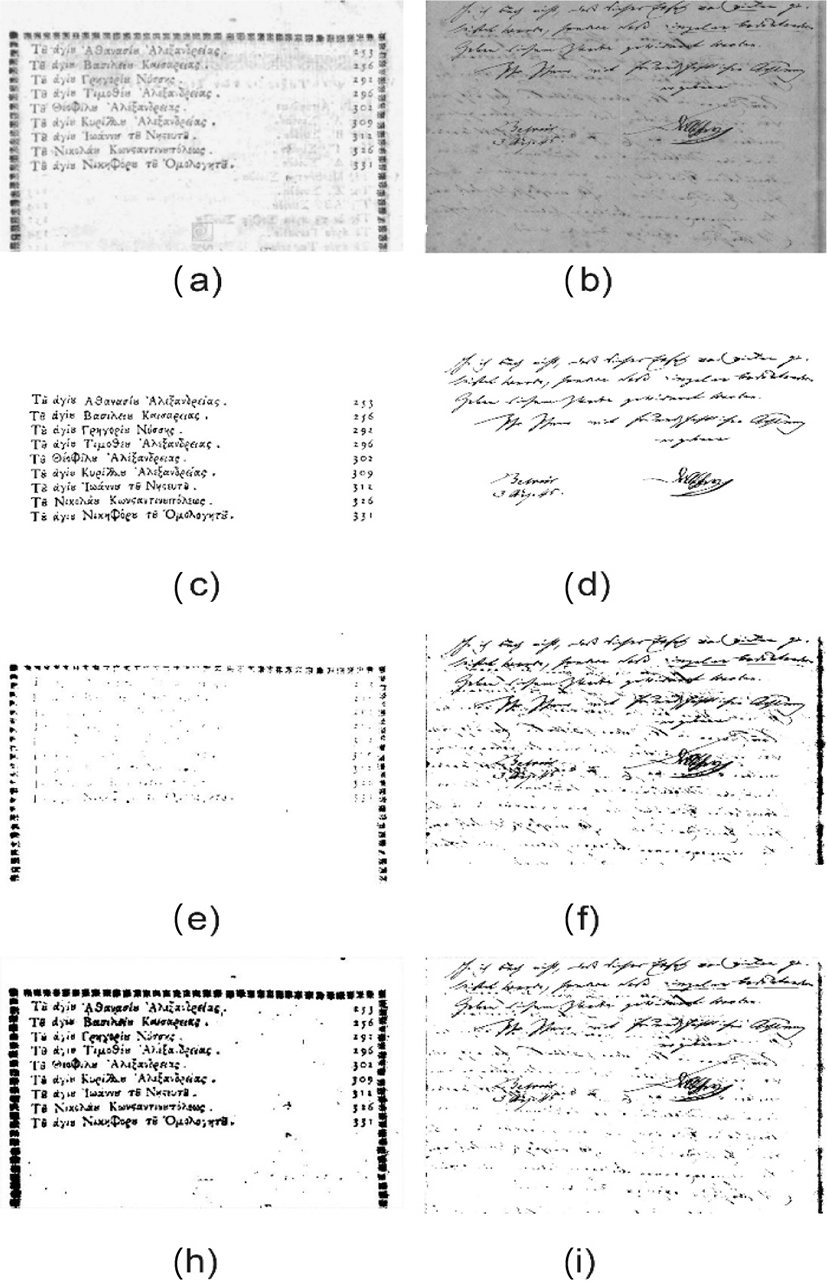Fig. 2. (a) and (b) are two images of DIBCO (2019) dataset; (c) and (d) are ground truth of them; (e) and (f) are result images of median-based thresholding on the original histogram; (h) and (i) are result images of median-based thresholding on the refined histogram.

In Fig. 3, two sample images (D34&D42) of the Brodatz dataset are thresholded by the Otsu method on their original and refined histograms. The images are in Fig. 3(a) and (b) and its ground truth masks are illustrated in Fig. 3(c) and (d).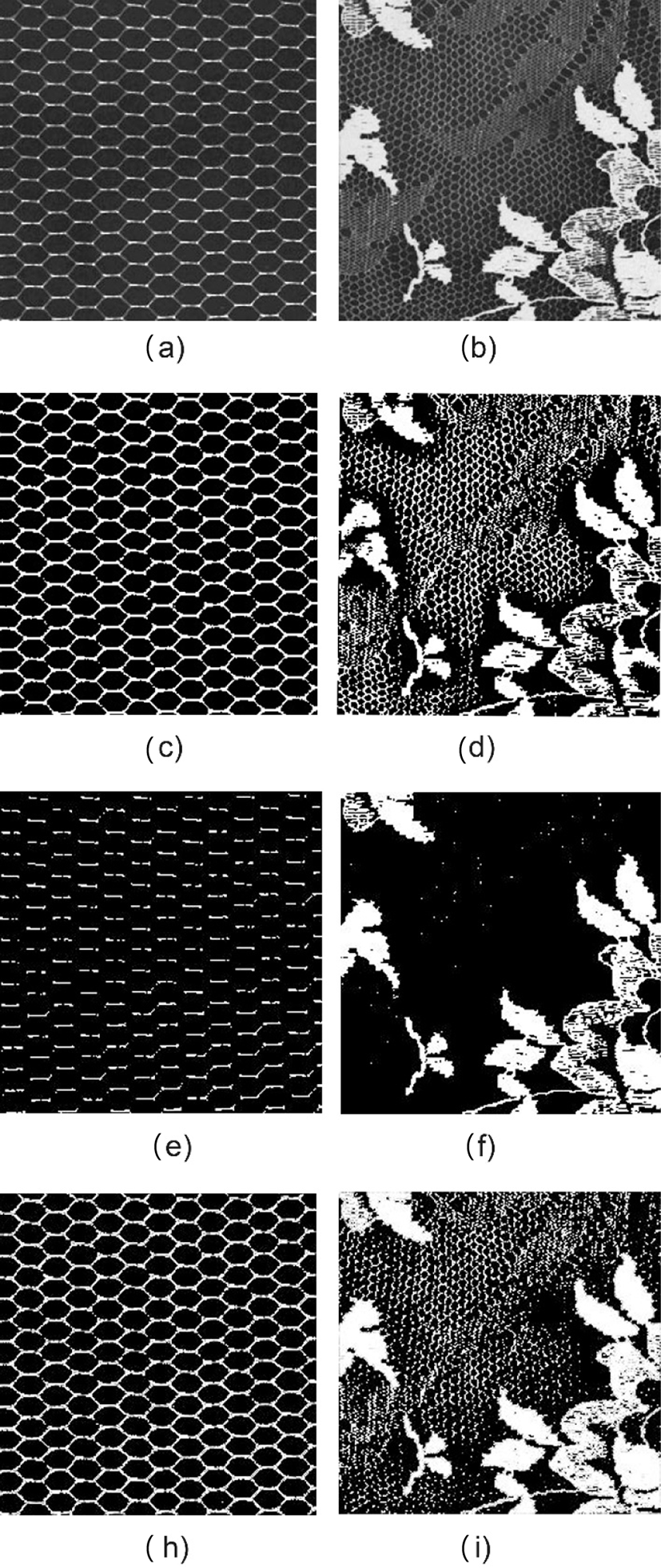Fig. 3. (a) and (b) are two images (D34 & D42) of Brodatz dataset; (c) and (d) are ground truth of them; (e) and (f) are result images of Otsu thresholding on the original histogram; (h) and (i) are result images of Otsu thresholding on the refined histogram.

Fig. 3(e) and (f) show the results of original histograms, and the mesh shown (e) has not clearly segmented compared to the refined histogram in Fig. 3(h). Similarly, Fig. 3 (i) shows the result of the refined histogram and it is better than the original one.

In Fig. 4, the Otsu and Median methods are used for segmenting, and their result and ground truth mask images are shown in Fig. 3(a)-(i), respectively. In Fig .4(e) and (f), both segmented results on original histograms are shown, and Fig. 4(h) and (i) are results of the refined histograms. The fishtail in Fig. 4(h) is the result of Otsu thresholding and it is better segmented in the refined histogram for comparison with the result of the original histogram Fig. 4(e). The screwdriver shown on the right bottom of Fig. 4 (i) is the result of the median-based and it is also better segmented than that of the original histogram Fig. 4 (f).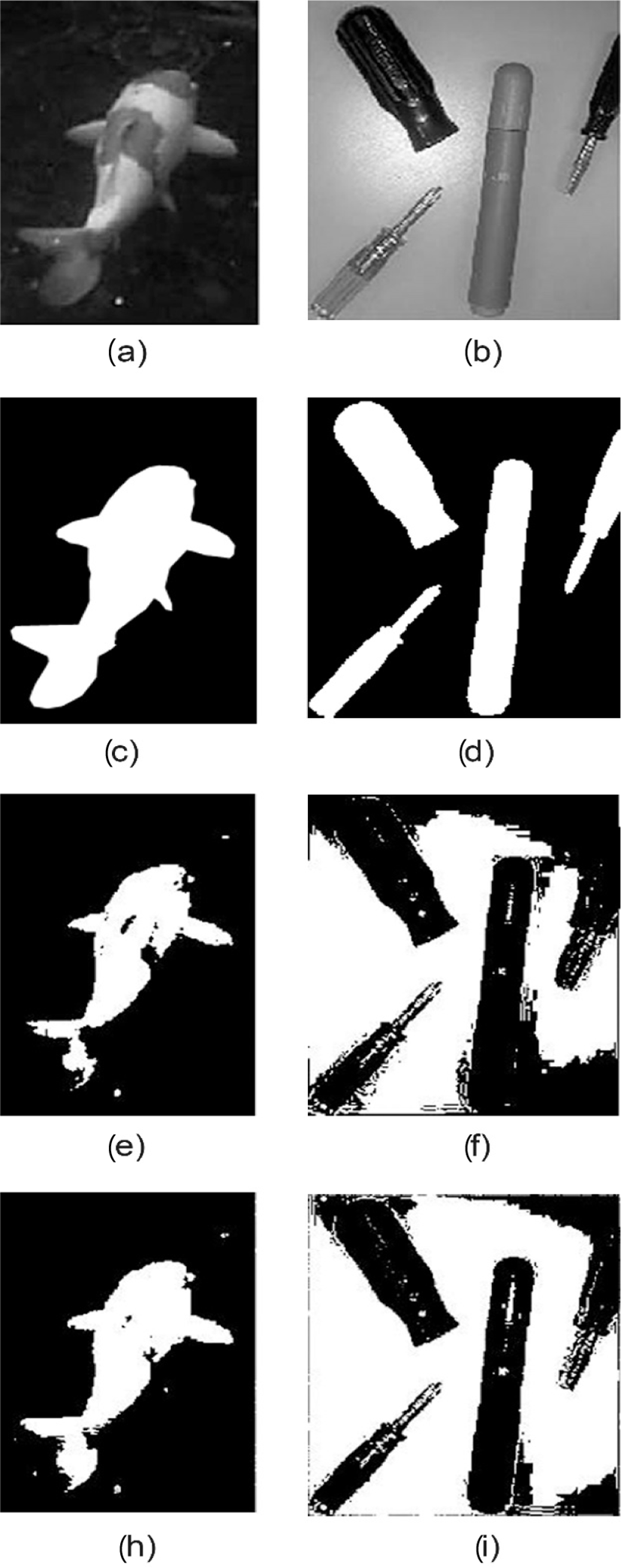Fig. 4. (a) fish and (b) screwdriver are original images; (c) and (d) are ground truth of them; (e) and (f) are result images of Otsu and median-based thresholding on the original histogram, respectively; (h) and (i) are result images of Otsu and median-based thresholding on the refined histogram, respectively.

Our experimental results prove that the results of them on the refined histograms are more effective compared with the original ones.

## V. CONCLUSION

We suggest the refined histogram for preprocessing of thresholding in order to reduce the big bin problem. In the work, we use Otsu and median-based thresholding techniques and six test images are chosen from DIBCO (2019) track A, Brodatz dataset, and others.

In order to reduce big-bin problem, we refined the histogram of the image using neighborhood distinction metric. Our experimental results prove that their results on the refined histograms are more effective compared with the original ones.

Further work will be to compare different thresholding methods’ results on a refined histogram using different neighborhood metrics.

## REFERENCES

.

M. Sezgin and B. Sankur, “Survey over image thresholding techniques and quantitative performance evaluation,” Journal of Electronic Imaging, vol. 13, no. 1, pp. 146-165, 2004..

N. R. Pal and D. Bhandari, “Image thresholding: Some new techniques,” Signal Processing, vol. 33, no. 2, pp.139-158, 1993..

C. A. Glasbey, “An analysis of histogram-based thresholding algorithms,” CVGIP: Graphical Models and Image Processing, vol. 55, no. 6, pp. 532-537, 1993..

S. Roy, P. Shivakumara, P. P. Roy, and C. L. Tan, “Wavelet-gradient-fusion for video text binarization,” in 21st International Conference on Pattern Recognition (ICPR 2012), Japan, Nov. 2012, pp. 3300-3303..

A. K. Bhunia, A. K. Bhunia, A. Sain, and P. P. Roy, “Improving document binarization via adversarial noise-texture augmentation,” in 2019 IEEE International Conference on Image Processing (ICIP), Taiwan, Sep. 2019, pp. 2721-2725..

T. R. Singh, S. Roy, O. I. Singh, T. Sinam, and K. Singh, “A new local adaptive thresholding technique in binarization,” IJCSI International Journal of Computer Science Issues, vol. 8, issue 6, no. 2, pp. 271-277, Nov. 2011..

N. Sengee and H. K. Choi, “Contrast enhancement using histogram equalization with a new neighborhood metrics,” Journal of Korea Multimedia Society, vol. 11, no. 6, pp. 737-745, Jun. 2008..

J. H. Xue and D. M. Titterington, “Median-based image thresholding,” Image and Vision Computing Volume, vol. 29, no. 9, pp. 631-637, Aug. 2011..

N. Otsu, “A threshold selection method from graylevel histograms,” IEEE Trans. Systems Man Cybernet, vol. 9, pp. 62-69, 1979..

.

A. Z. Arifina and A. Asano “Image segmentation by histogram thresholding using hierarchical cluster analysis,” Pattern Recognition Letters, vol. 27, no. 13, pp.1515-1521, Oct. 2006.## AUTHORS

Nyamlkhagva Sengeehas received his BS degree from National University of Mongolia in 2013 and his MS and Ph.D degrees in the Department of Computer Engineering from INJE University, Korea in 2008 and 2012, respectively. His research interests include medical image processing and analysis, contrast enhancement and image reconstruction algorithms.

Dalaijargal Purevsurenreceived his BS and MS degree from National University of Mongolia, in 2009 and 2011, respectively, and Ph.D in Computer Science from Harbin Institute of Technology, China in 2017. His interesting research areas are randomized algorithms and data mining.